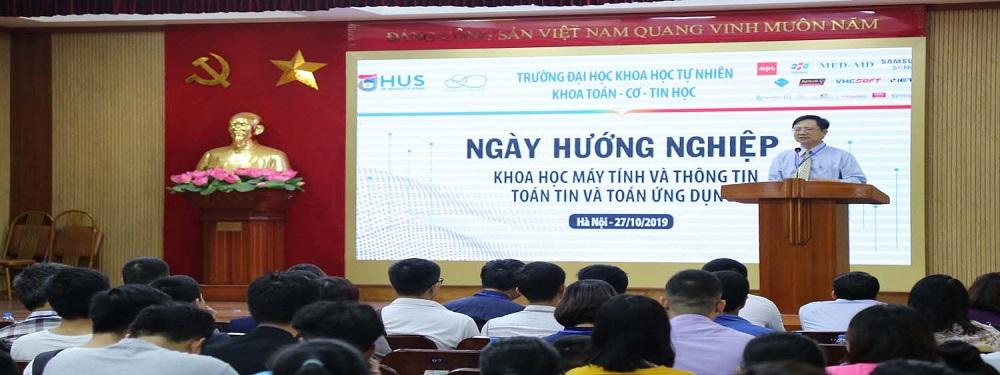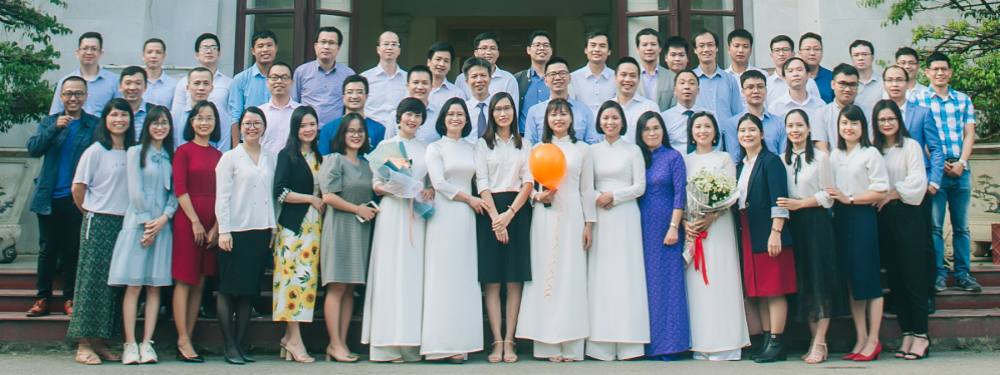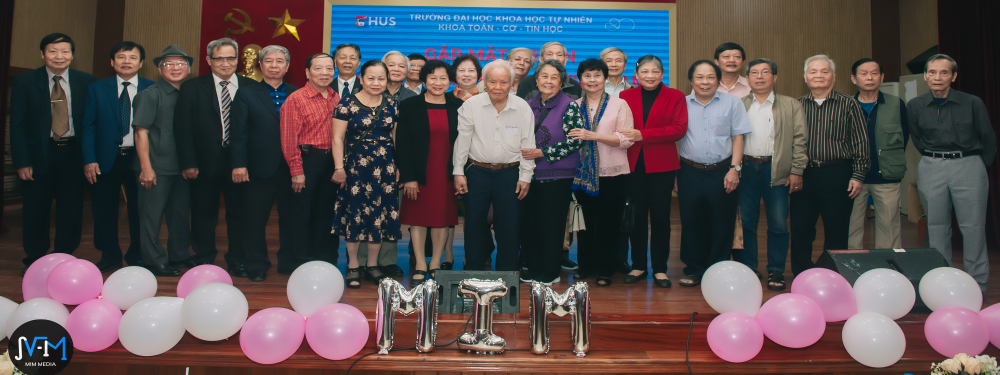EDUCATION

• Mathematics (standard and honor programs)
• Applied Mathematics and Informatics
• Computer and Information Science (standard and advanced programs)
• Data Science (open in 2020)

For graduate degree, there are 163 Master students and 76 PhD students specialized in:

• Algebra and Number Theory
• Analytical Mathematics
• Applied Mathematics
• Data Science (since 2018)
• Differential and Integral Equations (only PhD degree)
• Geometry - Topology
• Methods for Elementary Mathematics  (only Master degree)
• Solid Mechanics
• Fluid Mechanics
• Mathematical Foundations for Computer Science

INTRODUCTION

• Greetings
• Organization
• Development History